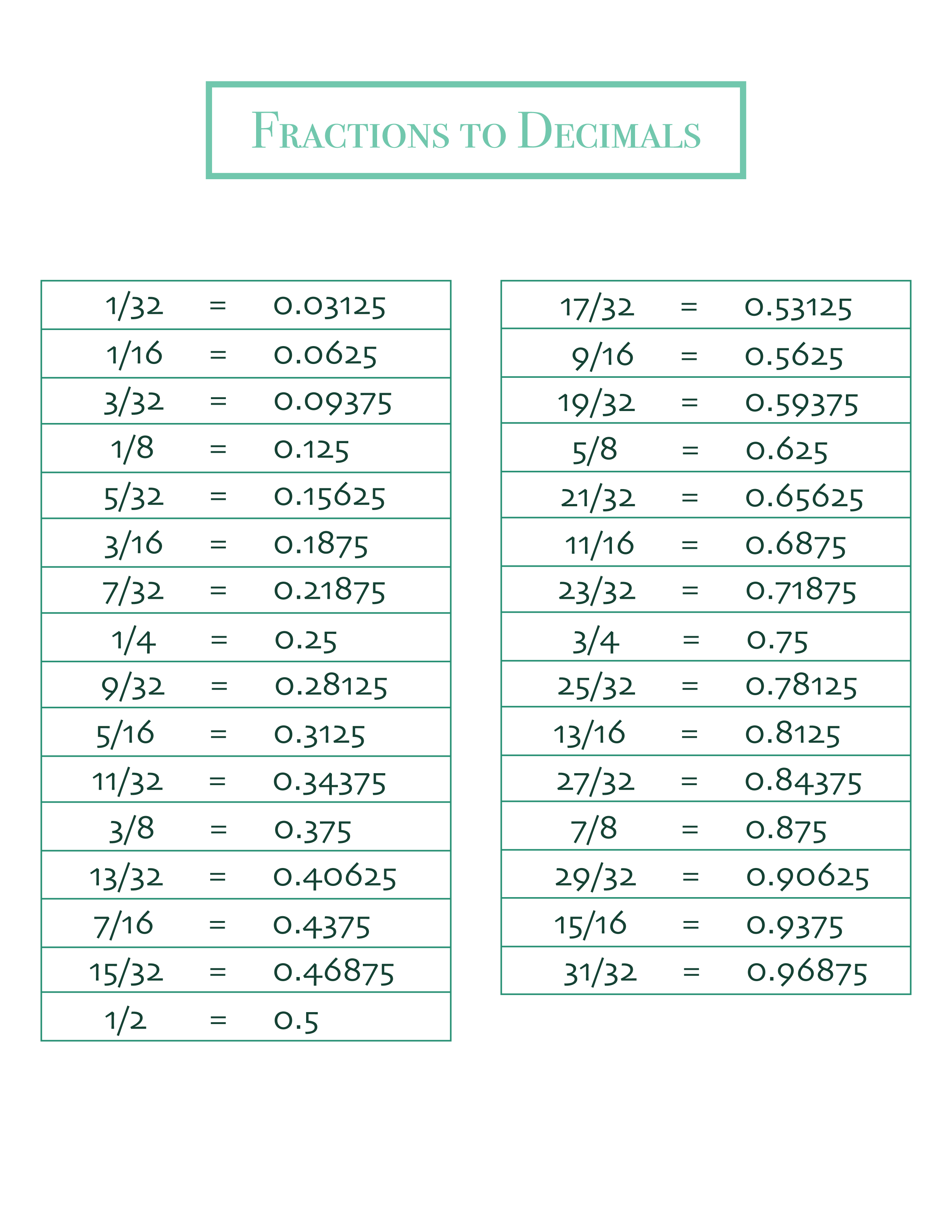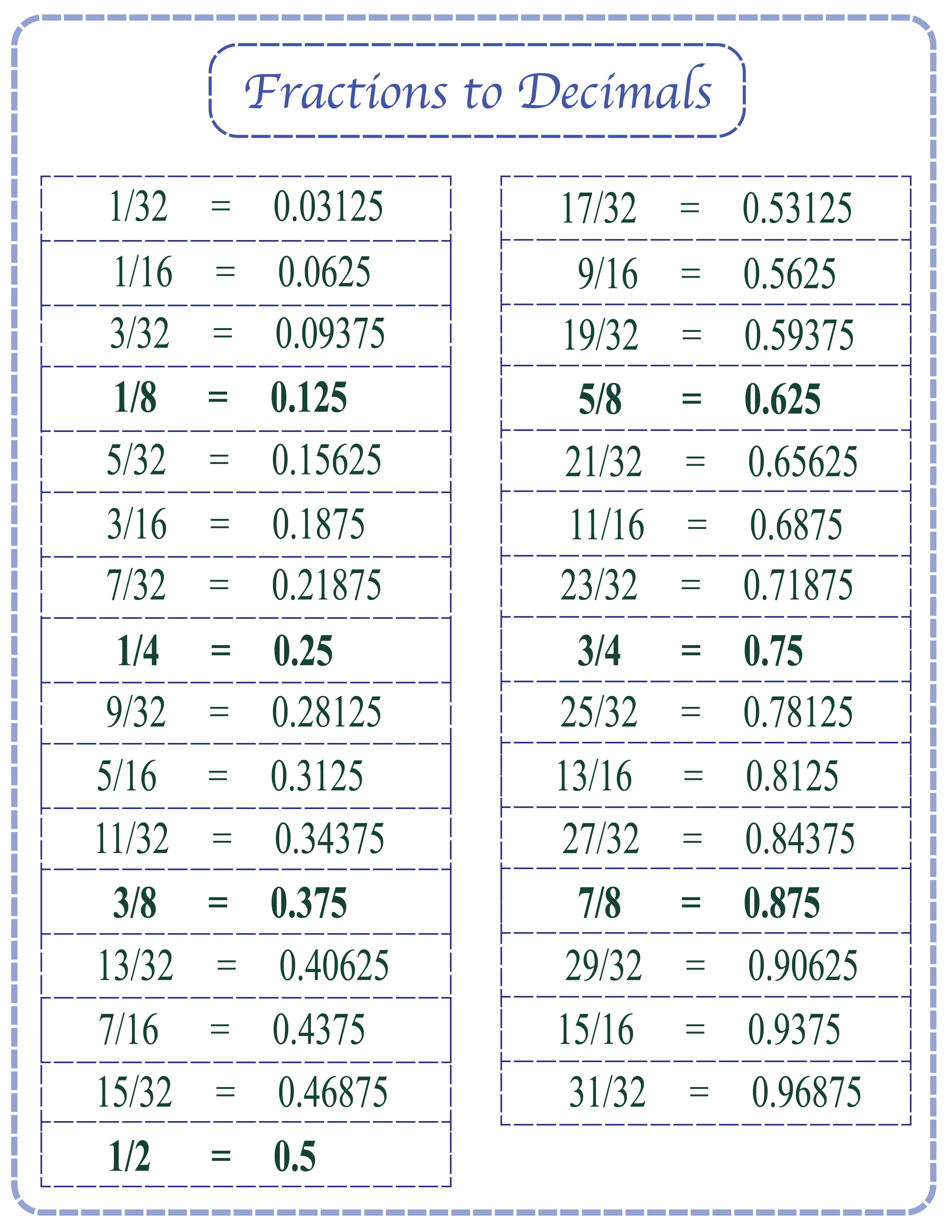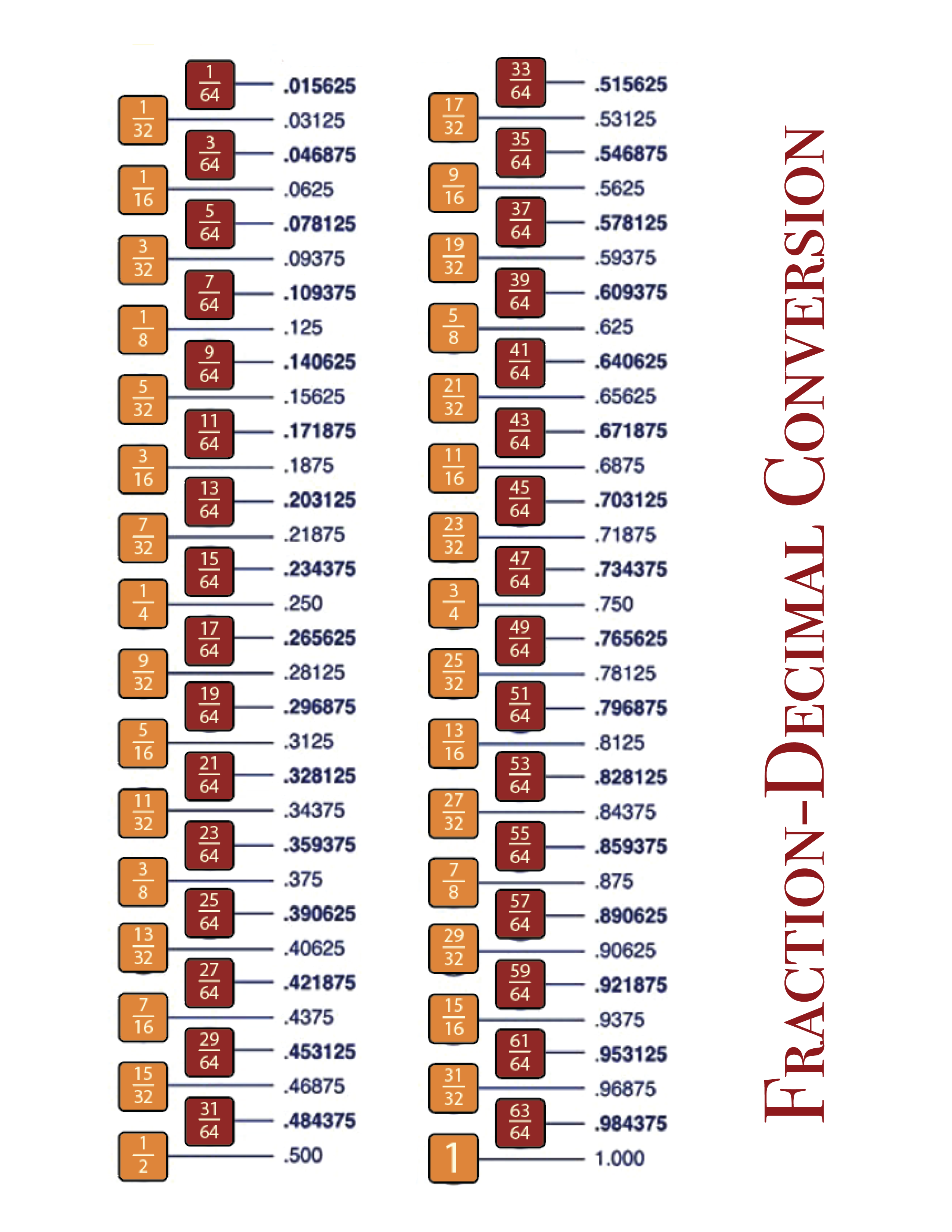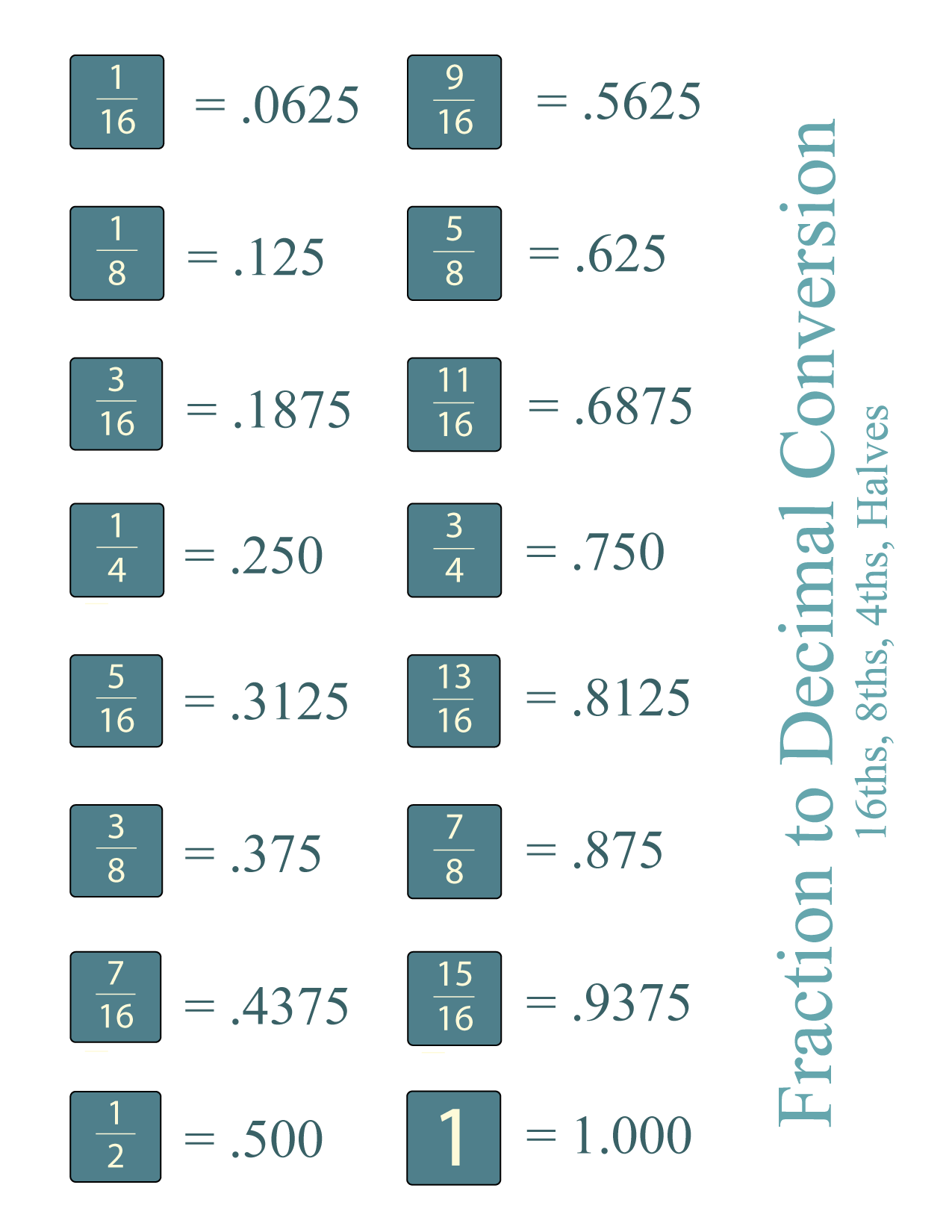Fractions and decimals are two ways of expressing parts of a whole, but it can be hard (especially for students) to remember how they relate to each other. In this post, we have free downloadable fraction to decimal conversion tables, both as images and PDFs.

If you need other printable conversion tables, check out our post here. We also have worksheets to practice adding and multiplying fractions.

## Fractions to decimals chart #1

Our first chart is both simple and comprehensive. It just has all the fractions on the left and the decimals on the right. This chart goes to 64ths, but scroll down for charts that focus on more common fractions.## Fractions to decimals chart #2

Some fractions are more common (and more important to memorize) than others. In this image, we have bolded those fractions and their decimal counterparts. If you are teaching a kid to memorize these conversions, the bolded numbers are the ones to start with!## Fraction to decimal conversion chart

The charts above can be a bit overwhelming with just blocks of numbers. In this post, we have differentiated between the 64ths on the right, and the larger fractions on the left.